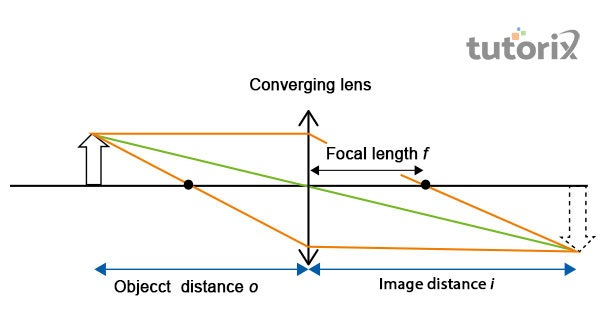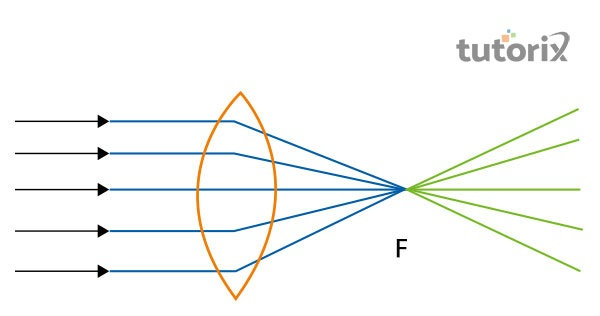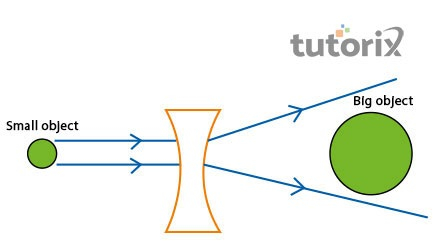# Thin Lens Formula for Concave and Convex Lenses

## Introduction

The thin lenses have the yields of a negative distance of image, and the virtual images are on the same side as the object faced on the lens. The positive and negative sides of the images are useful to calculate the distance of the image in the real life as a lens equation. The convention of the positive direction is always quantifiable with the direction where the light is travelling through the lens. The direction of the object is negative as the lens image travels a distance from the object and the direction is on the opposite side of the light travel.

## Thin Lens Formula

The radius curvature is negligible with the compared amount of lens’s thickness of a lens is a thin lens. The formula of a "thin lens" is used as f regarded as the "focal point", v regarded as the "distance from image", u regarded as the "distance from the object to the optical centre". Hence, the replicated formula will be

$$\mathrm{u\frac{1}{f}=\frac{1}{v}+\frac{1}{u}}$$

"The primary use case of the "concave lens" as the "negative focal length" is implicated in the length. On a contradictory note, the "positive focal point" of a lens is regarded as the "convex lens" (Hipólito et al. 2022). The calculation of the object’s distance is depending on the distance of the actual image is negative and may form a virtual image on the thin lens.Figure 1: "Equation of thin lens"

## Concave and Convex Lenses

The following section contains a detailed definition of the "Concave and Convex lenses":

### "Concave lens"

The lights get separated after falling on the "concave lenses" which is the reason; this lens has the most common use while driving. The surface of this type of lens is curved from the inside which allows forming both virtual and real images (Savini et al. 2020). The primary use of the "concave lens" is, that this can help identify the position and nature of the images based on the lens position. As this type of lens can make separation of lights, it is more useful to treat myopia (Hyperphysics, 2022). The utilisation of light can help in manipulating the light by utilising a piece of glass at a point, which is helpful to clear the blurry vision and make the object appear as clear.

### "Convex lens"

In a situation when the "spherical surfaces" are curved outside, the constriction of the "convex lens" happens, which is helpful for drivers to detect the distance of an object. These classes of lenses can consolidate the light coming from the outside and it helps to focus on a specific point to get a clear vision (Cinacchi & Torquato, 2019). The point is referred to as the focus (f).Figure 2: "Convex Lens"

## "Difference between Concave and Convex Lens"

The constructions of the "spherical lenses" are possible by joining two "surfaces of the spherical transparent". In the most common use case, there are two classes of "spherical lenses". Additionally, the "convex lenses" are basically constructed by connecting "the surfaces of two spherical" by protruding the exteriors, while the "concave lenses" are generally constructed by combining two exteriors of the spherical just like they are curved inside (Mori & Ogasawara, 2021). This is the primary distinction between "concave and convex lenses".

## Application of "Concave Lenses"

The followings are the practical application of the "Concave lenses":

### Telescope

The application of the "Convex lens" for constructing a telescope is possible due to the magnification of an object. However, to relax the eyepiece and focus on the entity more clearly, the application of the "Concave lens" is utilised.

### Eyeglass

The "concave lenses" are utilised in glasses which rectify the shortfall by circulating out the "light rays" before it contacts the eyeball. This allows an individual to see objects more clearly, which is placed far away. In order to treat myopia, this lens is taken into use.

### Peepholes

The panoramic view of an object placed outside of walls or doors is useful for using the peepholes on the door. This type of lens is proportional to minimise the object to get the whole view.

## Application of "Convex Lenses"

The followings are the application of the "Convex lenses":

### Camera

The camera lenses are consolidating the lights and the film is changed based on the utilisation. Moreover, the upcoming "rays of light" from the individual are connected by the "convex lens" constructing an image on the film or assessed couple machine in the matter of a "digital camera". Also, when the object is placed away from the lens and the light enters at a wider angle.

### "Magnifying Glass"

The magnified images can be produced with the application of the "convex lenses". The images are upright and virtual in the case of the "magnified images". The "virtual image" constructed is on the object’s identical side (Dewwool, 2022). For a "magnified image" to be celebrated the distance between the lens and the actual entity needs shorter than the lens’ "focal length".Figure 3: "Magnifying glass"

## Conclusion

Refraction is the essential principle of the optical devices with the utilisation of the glasses. Curved glasses are the primary classification of two different types as "concave and convex lenses". The thin centre of the edges is more usual in the conviction of the surfaces that are ideal in the situation where either the images are useful to treat myopia. The lenses are used in different situations that are either curved inside or outside. Moreover, based on the curve the reflection of light is used to make the use of the "focal length".

## FAQs

Q1. What is the distinction between "concave and convex lenses"?

Ans: The "concave lens" is thicker on the edges and the centre is thinner. However, in the case of a "convex lens," the edges are thinner whereas the centre is thicker.

Q2. Which objects are connected to considering the formula of the thin lens?

Ans: The relation between the distance of image, object, and the "focal point" of a lens is related to the determination of a thin lens.

Q3. What is the application of "concave lenses"?

Ans: Different use cases of the "concave lens" in daily life are: "eyeglasses, telescope, and binoculars".

Updated on: 13-Oct-2022

532 Views